# Solving the Non-Damped Oscillatory Problem with Random Loading Conditions using WHE Technique

Amnah S Al Juhani1* and Aisha M Al Hwiti2

1Tabuk University, Faculty of Science, Saudi Arabia

2Tabuk University, Faculty of Science, Saudi Arabia

Received Date: June 10 , 2019;  Published Date: June 17 , 2019

#### Abstract

The spectral techniques for solving the stochastic differential equations (SDEs) are efficient compared with other techniques. They can be used to obtain analytical solutions specially in case of linear SDEs. Among which, the Wiener-Hermite expansion (WHE) and the Winer Chaos expansion (WCE) are the most common. WHE is more efficient as it depends directly on a basis that is directly dependent on the noise process. It can be used efficiently when the SDE is forced by additive and/or multiplicative noise.

In the current work, WHE is used to analyze the stochastic non-damped oscillatory equation. The corresponding deterministic system is obtained, and solution techniques are suggested and compared. The solution statistics are computed in analytical formulae and compared for different parameters. The efficiency of WHE is outlined as a reliable technique that can be used for any order of approximation in linear and nonlinear SDEs.

Keywords:Linear stochastic differential equations; Wiener-hermite expansion; Linear oscillatory; WHEP technique; Mathematical

#### Introduction

Since Meecham and his co-workers  developed a theory of turbulence involving a truncated Wiener-Hermite expansion (WHE) of the velocity field, many authors studied problems concerning turbulence. Various applications in fluid mechanics was also studied in [2-4]. Scattering problems attracted the WHE applications through many authors. The nonlinear oscillators were considered as an opened area for the applications of WHE as can be found in [5-10]. There are a lot of applications in boundary value problems and generally in different mathematical studies.

The Wiener Hermite Expansion (WHE) was originally developed by Norbert Wiener in 1938-1958 Wiener constructed an orthonormal random bases for expanding homogeneous chaos depending on white noise and used it to study problems in statistical mechanics]. In (WHE) approach, there is no randomness directly involved in the computations, one does not have to rely on pseudo random number generators, and there is no need to solve the stochastic DEs repeatedly for many realizations [11-20].

Instead, the deterministic system is solved only once by converting the problem to a system of deterministic equations that can be solved efficiently using the standard deterministic numerical methods. After that it is easy to determine mean, covariance, and higher order statistical moments, by using simple formulae involving only the deterministic Wiener-Hermite coefficients. The application of WHE aims at finding a truncated series solution of differential equations . The truncated series composes of two major parts, the first is the Gaussian part which consists of the first two terms, while the rest of the series constitute the non-Gaussian part. When solving nonlinear stochastic integrodifferential equations using the WHE, the resultant set of deterministic integrodifferential equations obtained are quite complicated and it is not always possible to find an analytic solution.

#### Problem Formulation

The main objective of this paper is to solve the following linear oscillatory problem without damping with random loading condition .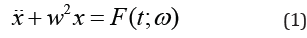with deterministic initial conditionsWhere w: frequency of oscillation, and the stochastic excitation F (t, ω ) is defined as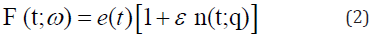where

ε is the deterministic nonlinearity scale,

ω ∈(Ω,σ , P) is the triple probability space with Ω as the sample space,

σ is a σ -algebra on the event space Ω ,

P is a probability measure, and

n (t;ω) is white noise with the following properties: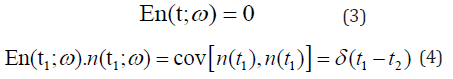In the following section we will find the probability density function (p.d.f) average and the variance for x(t).

#### Finding the P.d.f, Average and Variance of x (t):

The general solution of equation (1) is,Due to linearity and the deterministic initial conditions we obtain the following Gaussian solution process:Equation (9) represent a closed form solution of problem (1) with random loading condition.

#### WHEP Technique

The application of the WHE aims at finding a truncated series solution to the solution process of differential equations. The truncated series composes of two major parts; the first is the Gaussian part which consists of the first two terms, while the rest of the series constitute the non-Gaussian part. In nonlinear cases, there exists always difficulties of solving the resultant set of deterministic integrodifferential equations got from the applications of a set of comprehensive averages on the stochastic integra-differential equation obtained after the direct application of WHE. Many authors introduced different methods to face these obstacles. Among them, the WHEP technique was introduced in  using the perturbation technique to solve perturbed nonlinear problems.

The WHE method utilizes the Wiener-Hermite polynomials which are the elements of a complete set of statistically orthogonal random functions . The Wiener-Hermite polynomial H(i) (t1,t2,t3,…ti) satisfies the following recurrence relation: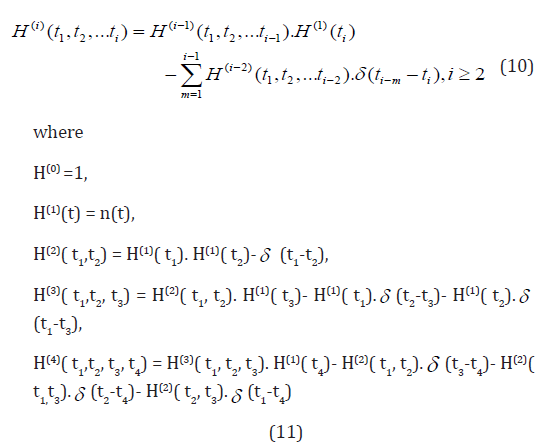in which n(t) is the white noise with the following statistical properties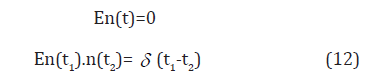where δ (-) is the Dirac delta function and E denotes the ensemble average operator

The Wiener-Hermite set is a statistically orthogonal set, i.e.The average of almost all H functions vanishes, particularly,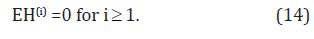Due to the completeness of the Wiener-Hermite set, any random function G(t;ω ) can be expanded as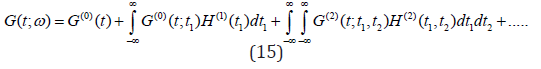where the first two terms are the Gaussian part of G(t;ω ). The rest of the terms in the expansion represent the non-Gaussian part of G(t;ω ). The average of G(t; ω ) isThe WHEP technique can be applied to linear or nonlinear perturbed systems described by ordinary or partial differential equations. The solution can be modified in the sense that additional parts of the Wiener-Hermite expansion can always be taken into considerations and the required order of approximations can always be made depending on the computing tool. It can be even run through a package if it is coded in some sort of symbolic languages. The technique was successfully applied to several nonlinear stochastic equations, see [20-22].

#### Case Study [23-26]

In the previous case of study solve by using WHEP technique.

The following results are obtained:To compensate by the original formula of (10) we get:Accordingly, we obtain the following:It can be show that: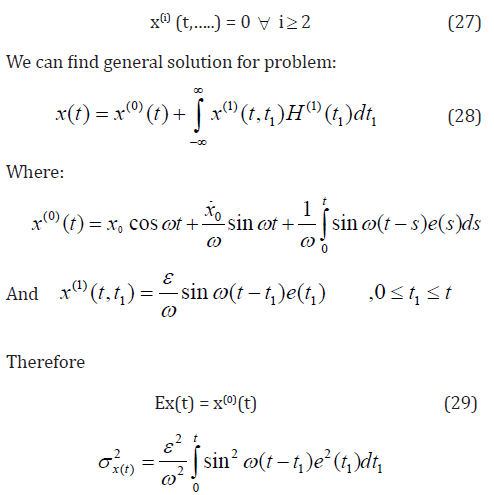This method is more reliable than others, since we do not have the perturbation problem because the Wiener-Hermite expansion belong to a complete space.

Example:

Consider the followingIn the case-study presented in section 4, the following results are obtained (Figures 1-8):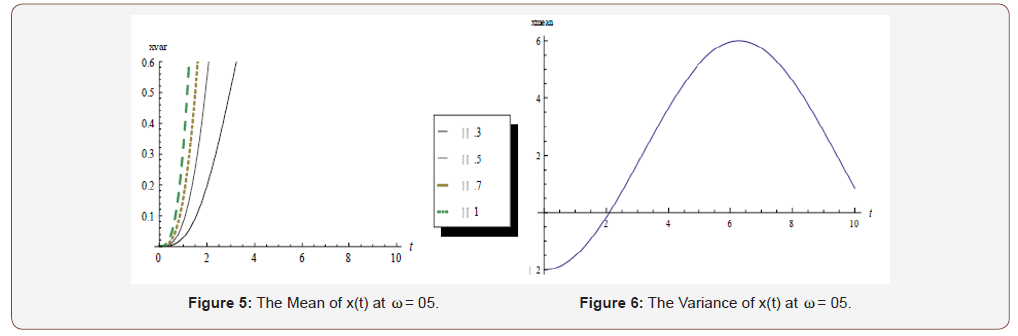#### Conclusion

By comparing the solution that we get using this method with the one obtained using the WHEP technique, we found that the two solutions are identical. Where we have considered the Gaussian part only in this comparison. The WHEP technique is a combination of the homotropy perturbation method and the wiener-Hermite technique.

None.

#### Conflict of Interest

No conflict of interest.

Article Details
Citation
Keywords
Scroll to
Scroll to Top